Latest Teaching jobs   »   CTET 2015 Maths Pedagogy Questions: Free...

# CTET 2015 Maths Pedagogy Questions: Free PDF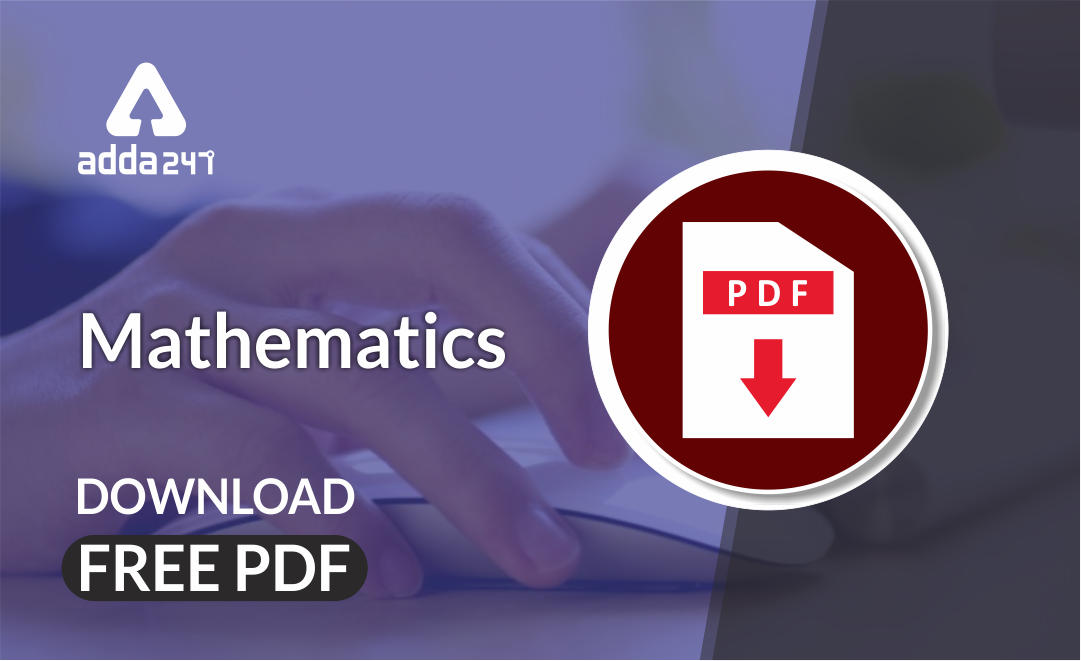Mathematics is an equally important section for CTET, MPTET, KVS & DSSSB Exams and has even more abundant importance in some other exams conducted by central or state govt.

To let you make the most of Mathematics section, we are providing important previous year Maths Pedagogy Questions.  We wish you all the best of luck to come over the fear of the Mathematics section.

Mathematics Study Notes For All Teaching Exams

## CTET 2015 (Paper 2) Maths Pedagogy Questions

Q1. The term, ‘Mathematical tools’ refers to

(a) Charts based on formulae and concepts, graph papers, dotted sheets, etc.

(b) Calculators, rulers, tape measures, protractors, compass, etc.

(c) All types of materials including language, written symbols, meaningful instructions to

establish their purpose

(d) Physical material like geo – board and 3D models, cubic rods, etc.

Q2. In class VI, in the unit of “Understanding Quadrilaterals’, important results related to angle – sum property of quadrilaterals are introduced using paper folding activity followed by the exercise based on these properties. At this level proof of the angle property is not given, as the students of class VI are at Van Hiele level of

(a) Level 1 – Analysis

(b) Level 2 – Informal Deduction

(c) Level 3 – Deduction

(d) Level – Visualization

Q3. Which one of the following statements is correct?

(a) ‘1’ is the smallest prime number.

(b) Sum of two prime numbers is always a prime number.

(c) A composite number can be odd.

(d) There is no even prime number

Q4. Read the following question from class VI textbook: “Write a pair of integers whose sum gives negative integer.” The above question refers to

(a) Closed – ended question

(b) Reflective question

(c) Multi – disciplinary question

(d) Open – ended question

Q5. CBSE announced the celebration of ‘GANIT Week’ in schools to commemorate the birth anniversary of the legendary mathematician, Srinivasa Ramanujan. GANIT stands for

(a) Growing Aptitude in Numerical Innovations and Training

(b) Growing Ability in Numerical Innovations and Techniques

(c) Growing Ability in Numerical Innovations and Training

(d) Growing Aptitude in Numerical Innovations and Techniques.

Q6. A learner exhibiting difficulty in sorting, recognizing patterns, orienting numbers and shapes, telling time and measurement may have dyscalculia with difficulty in

(a) visual – memory

(b) language processing

(c) visual – motor coordination

(d) visual – spatial skills

Q7. Four stages of language development in mathematics classroom in order are

(a) Everyday language, → Symbolic language → Language of Mathematics problem solving → Mathematized situation language

(b) Everyday language, → Mathematized situation language → Language of Mathematics problem solving → Symbolic language

(c) Everyday language, → Language of Mathematics problem solving → Mathematized situation language → Symbolic language

(d) Everyday language, → Language of Mathematics problem solving → Symbolic language → Mathematized situation language

Q8. Learning mathematics at upper primary level is about

(a) learning problem solving techniques only.

(b) gaining understanding of mathematical concepts and their applications in solving problems logically

(c) learning lots of new formulae and algorithms.

(d) remembering solutions or methods of various types of mathematical problems

Q9. In geometry class of VI grade students, the teacher explained the construction of angles measuring 30°, 60° and 90°, with the help of demonstration of construction and bisector of an angle. Then she asked the students to construct an angle of 15° and an angle of 45°. This task at this point reflects the teacher’s intention to

(a) give the exposure of experiential learning.

(b) assess the learner’s performance in summative assessment.

(c) assess the student’s understanding and ability to combine two skills learnt, to accomplish the given task.

(d) engage every student in some work.

Q10. Place of mathematics education in the curricular framework is positioned on twin concerns

(a) What mathematics can do to retain every child in school and how it can help them to be self – dependent

(b) What mathematics education can do to improve the score of students in summative

examination and how it can help to choose right stream in higher classes

(c) What mathematics education can do to improve communication skills of every child and how it can make them employable after school

(d) What mathematics education can do to engage the mind of every student and how it can strengthen the student’s resources

Mathematics Quiz For CTET Exam : Attempt Daily Quizzes

Q11. As per NCF 2005, the goal of mathematics teaching in school curriculum is that children learn “Important Mathematics”. Important Mathematics implies

(a) solving mathematical games and puzzles.

(b) understanding appropriate use of learnt mathematical techniques

(c) verifying geometrical theorems in Maths Lab.

(d) knowing mathematical procedures and algorithms

Q12. ‘Data Handling’ at upper primary stage focuses on:

(a) data interpretation only

(b) data organization only

(c) techniques of data collection

(d) data collection, organization and interpretation

Q13. Which one of the following is not a Problem-Solving Strategy in Mathematics?

(a) Drawing

(b) Solving backwards

(c) Rote learning

(d) Trial and Error

Q14. According to Piaget, when the child is at formal operational stage, it is appropriate to introduce:

(a) Data handling

(b) Numbers

(c) Geometry

(d) Ratio and Proportion

Q15. Read the following problem given in a textbook for class VII: “A map is given with a scale of 2 cm = 1000 km. What is the actual distance between two places, in kms, if the distance on the map is 2.5 cm? This problem is:

(a) Investigatory in nature.

(b) based on higher order thinking skills.

(c) to enhance problem solving skills.

(d) Interdisciplinary in nature.

Q16. Which one of the following is not a Mathematical process?

(a) Estimation

(b) Memorization

(c) Measurement

(d) Visualization

Q17. According to NCF 2005, school Mathematics takes place in a situation where:

(a) children are forced to learn all concepts by daily practice.

(b) children are listeners and the teacher is an active narrator.

(c) children are involved in chorus drill of formulae and pressure of performance in

examination.

(d) mathematics is part of children’s life experience

Q18. In class VI, teacher gave a topic for debate as Formative Assessment (FA) task. “Hindu – Arabic numerals are more powerful than Roman Numerals. “Objective of this FA activity is to assess

(a) Analysis

(b) Creativity

(c) Understanding

(d) Knowledge

Q19. In a Mathematics classroom, emphasis is placed on:

(a) mathematical content, process and reasoning

(b) problem solving strategies

(c) mathematical algorithms and processes

(d) mathematical content

Q20. A teacher gives the following problem related to ‘discount’ to the students for solving. “The Marked Prices (M.P.) of a shirt and sweater are Rs. 200 and Rs. 300 respectively, and the discounts on the marked prices of shirt and sweater are 20% and 12% respectively. While preparing the bill, the shopkeeper interchanged the discounts on these items by mistake. On getting the bill, Hamida noticed the mistake and paid the actual amount to the shopkeeper. How much money did Hamida pay to the shopkeeper?” What value does the teacher try to promote through this problem?

(a) Honesty

(b) Co – operation

(c) Sympathy

(d) Compassion

Solutions
S1. Ans.(c)

Sol. All types of materials including language, written symbols, meaningful instructions to establish their purpose

S2. Ans.(b)

Sol. In mathematics education, the Van Hiele model is a theory that describes how students learn geometry.

The best-known part of the Van Hiele model are the five levels which the Van Hieles postulated to describe how children learn to reason in geometry.

Level 2: Informal Deduction

At this level, students can establish the interrelationship of properties both within figures (e.g., in a quadrilateral, opposite sides being parallel necessitates opposite angles being equal) and among figures (a square is a rectangle because it has all the properties of a rectangle). Thus, they can deduce properties of a figure and recognize classes of figures. Class inclusion is understood. Definitions are meaningful. Informal arguments can be followed and given. The student at this level, however, does not comprehend the significance of deduction as a whole or the role of axioms.

S3. Ans.(c)

Sol. A composite number can be odd e.g.15 = 5 × 3

Hence, 15 is odd number

S4. Ans.(d)

Sol. Open – ended Questions seeks from respondents to answer in their own words. Here answers are not unique and fixed.

S5. Ans.(a)

Sol. GANIT stands as an acronym for Growing Aptitude Innovations & Training.

S6. Ans.(c)

Sol. Dyscalculia is difficulty in learning or comprehending arithmetic, such as difficulty in understanding numbers, learning how to manipulate numbers, and learning facts in mathematics. It is generally seen as a specific developmental disorder.

S7. Ans.(b)

Sol. Everyday language, → Mathematized situation language → Language of Mathematics problem solving → Symbolic language

S8. Ans.(b)

Sol. Learning mathematics at upper primary level is about gaining understanding of mathematical concepts and their applications in solving problems logically.

S9. Ans.(c)

Sol. The given task assesses the student’s understanding and ability to combine two skills learnt, to accomplish the given task.

S10. Ans.(d)

Sol. What mathematics education can do to engage the mind of every student and how it can strengthen the student’s resources.

S11. Ans.(b)

Sol. A VISION STATEMENT: NCF – 2005 envisioned that, school mathematics takes place in a situation where

• Children learn to enjoy mathematics:
• Children learn important mathematics:
• Children see mathematics as something to talk about, to communicate, to discuss among themselves to work together on.
• Children pose and solve meaningful problems: In school, mathematics is the domain, which formally addresses problem solving as a skill.
• Children use abstractions to perceive relationships, to see structure, to reason about things, to argue the truth or falsity of statements.
• Children understand the basic structure of mathematics: Arithmetic, algebra, geometry and trigonometry etc.
• Teachers expect to engage very child in class

S12. Ans.(d)

Sol. Data handling is one of the central activities in which real mathematicians engage: students are frequently analysing data that they have gathered in various contexts and looking for patterns and generalities within them.

S13. Ans.(c)

Sol. Rote learning is a memorization technique based on repetition. The idea is that one will be able to quickly recall the meaning of the material the more one repeats it. Teaching children maths by rote could worsen exam results because they risk failing to properly understand the subject.

S14. Ans.(d)

Sol. The formal operational stage begins at about age 11. As students enter this stage, they gain the ability to think in an abstract manner, the ability to combine and classify items in a more sophisticated way, and the capacity for higher – order reasoning. At about age 11+ years, the child begins to manipulate ideas in its head, without any dependence on concrete manipulation, it has entered the formal operational stage. He/she can do mathematical calculations, think creatively, use abstract reasoning, and imagine the outcome of particular actions.

S15. Ans.(d)

Sol. Interdisciplinary approach is a technique or tool that establishes reciprocal relationship for better understanding of the subject.

S16. Ans.(b)

Sol. The act of memorisation is often a deliberate mental process undertaken in order to store in memory for later recall items such as experiences, names, appointments, addresses, telephone numbers, lists, stories, poems, pictures, maps, diagrams, facts, music or other visual, auditory, or tactical information. Memorisation is not a Mathematical process.

S17. Ans.(d)

Sol. NCF 2005 emphasises that school learning should not be confined to textbooks alone; teaching – learning experiences should be embedded in the child’s life experiences. For this, it is necessary that learning should be shifted form rote method. This clearly requires that the school system should be flexible, allowing innovation and promoting creativity among children. Overall development of the child should be emphasized.

S18. Ans.(a)

Sol. Analysis refers to the ability to break down material into its component parts so that its organizational structure may be understood.

S19. Ans.(a)

Sol. In a Mathematics classroom, emphasis is placed on mathematical content, process and reasoning.

S20. Ans.(a)

Sol. By paying actual amount Hamida shows her honesty. As an honest person doesn’t do things that are morally wrong.

•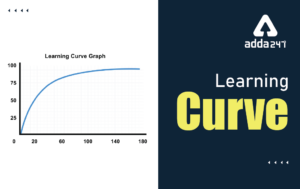Learning Curve - What is Learning Curve?...
•रस - परिभाषा, भे�...
•पठन कौशल- परिभा�...
•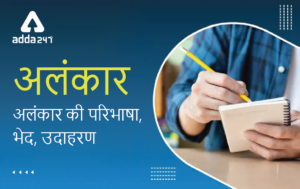अलंकार - परिभाष�...
•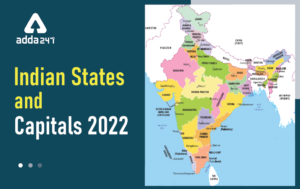Indian States and Capitals 2022: 28 Stat...
•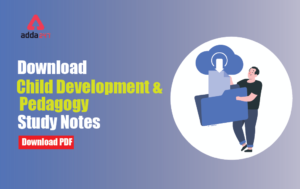New Education Policy(NEP) PDF - नई �...